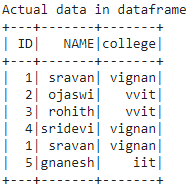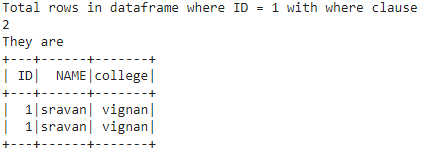Related Articles

# Count rows based on condition in Pyspark Dataframe

• Last Updated : 29 Jun, 2021

In this article, we will discuss how to count rows based on conditions in Pyspark dataframe.

For this, we are going to use these methods:

• Using where() function.
• Using filter() function.

Creating Dataframe for demonstration:

## Python3

 `# importing module``import` `pyspark`` ` `# importing sparksession from pyspark.sql module``from` `pyspark.sql ``import` `SparkSession`` ` `# creating sparksession and giving an app name``spark ``=` `SparkSession.builder.appName(``'sparkdf'``).getOrCreate()`` ` `# list  of students  data ``data ``=``[[``"1"``,``"sravan"``,``"vignan"``],``       ``[``"2"``,``"ojaswi"``,``"vvit"``],``       ``[``"3"``,``"rohith"``,``"vvit"``],``       ``[``"4"``,``"sridevi"``,``"vignan"``],``       ``[``"1"``,``"sravan"``,``"vignan"``], ``       ``[``"5"``,``"gnanesh"``,``"iit"``]]`` ` `# specify column names``columns ``=` `[``'ID'``,``'NAME'``,``'college'``]`` ` `# creating a dataframe from the lists of data``dataframe ``=` `spark.createDataFrame(data,columns)`` ` `print``(``'Actual data in dataframe'``)``dataframe.show()`

Output:Note: If we want to get all row count we can use count() function

Syntax: dataframe.count()

Where, dataframe is the pyspark input dataframe

Example: Python program to get all row count

## Python3

 `print``(``'Total rows in dataframe'``)``dataframe.count()`

Output:

```Total rows in dataframe
6```

### Method 1: using where()

where(): This clause is used to check the condition and give the results

Syntax: dataframe.where(condition)

Where the condition is the dataframe condition

Example 1: Condition to get rows in dataframe where ID =1

## Python3

 `# condition to get rows in dataframe ``# where ID =1``print``('Total rows ``in` `dataframe where\``ID` `=` `1` `with where clause')``print``(dataframe.where(dataframe.``ID` `=``=` `'1'``).count())`` ` `print``(``'They are  '``)``dataframe.where(dataframe.``ID` `=``=` `'1'``).show()`

Output:Example 2: Condition to get rows in dataframe with multiple conditions.

## Python3

 `# condition to get rows in dataframe``# where ID not equal to 1``print``('Total rows ``in` `dataframe where\``ID` `except` `1` `with where clause')`` ` `print``(dataframe.where(dataframe.``ID` `!``=` `'1'``).count())`` ` `# condition to get rows in dataframe``# where college is equal to vignan``print``('Total rows ``in` `dataframe where\``college ``is` `vignan with where clause')``print``(dataframe.where(dataframe.college ``=``=` `'vignan'``).count())`` ` ` ` `# condition to get rows in dataframe``# where id greater than 2``print``('Total rows ``in` `dataframe where ``ID` `greater\``than ``2` `with where clause')``print``(dataframe.where(dataframe.``ID` `> ``2``).count())`

Output:

Total rows in dataframe where ID except 1 with where clause

4

Total rows in dataframe where college is vignan with where clause

3

Total rows in dataframe where ID greater than 2 with where clause

3

Example 3: Python program for multiple conditions

## Python3

 `# condition to get rows in dataframe``# where ID not equal to 1 and name is sridevi``print``('Total rows ``in` `dataframe where ``ID` `\``not` `equal to ``1` `and` `name ``is` `sridevi')``print``(dataframe.where((dataframe.``ID` `!``=` `'1'``) &``                      ``(dataframe.NAME ``=``=` `'sridevi'``)``                     ``).count())`` ` `# condition to get rows in dataframe``# where college is equal to vignan or iit``print``('Total rows ``in` `dataframe where college ``is``\``vignan ``or` `iit with where clause')``print``(dataframe.where((dataframe.college ``=``=` `'vignan'``) |``                      ``(dataframe.college ``=``=` `'iit'``)).count())`

Output:

Total rows in dataframe where ID not equal to 1 and name is sridevi

1

Total rows in dataframe where college is vignan or iit with where clause

4

### Method 2: Using filter()

filter(): This clause is used to check the condition and give the results, Both are similar

Syntax: dataframe.filter(condition)

Example 1: Python program to get rows where id = 1

## Python3

 `# condition to get rows in``# dataframe where ID =1``print``('Total rows ``in` `dataframe where\``ID` `=` `1` `with ``filter` `clause')``print``(dataframe.``filter``(dataframe.``ID` `=``=` `'1'``).count())`` ` `print``(``'They are  '``)``dataframe.``filter``(dataframe.``ID` `=``=` `'1'``).show()`

Output:Example 2: Python program for multiple conditions

## Python3

 `# condition to get rows in dataframe``# where ID not equal to 1 and name is sridevi``print``('Total rows ``in` `dataframe where ``ID` `not``\``equal to ``1` `and` `name ``is` `sridevi')``print``(dataframe.``filter``((dataframe.``ID` `!``=` `'1'``) &``                       ``(dataframe.NAME ``=``=` `'sridevi'``)).count())`` ` `# condition to get rows in dataframe``# where college is equal to vignan or iit``print``('Total rows ``in` `dataframe where college\``is` `vignan ``or` `iit with ``filter` `clause')``print``(dataframe.``filter``((dataframe.college ``=``=` `'vignan'``) |``                       ``(dataframe.college ``=``=` `'iit'``)).count())`

Output:

Total rows in dataframe where ID not equal to 1 and name is sridevi

1

Total rows in dataframe where college is vignan or iit with filter clause

4

Attention geek! Strengthen your foundations with the Python Programming Foundation Course and learn the basics.

To begin with, your interview preparations Enhance your Data Structures concepts with the Python DS Course. And to begin with your Machine Learning Journey, join the Machine Learning – Basic Level Course

My Personal Notes arrow_drop_up